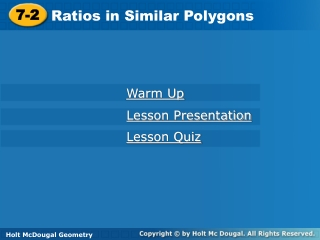Download Presentation7-2# 7-2 - PowerPoint PPT Presentation

Download Presentation##### 7-2

Download Policy: Content on the Website is provided to you AS IS for your information and personal use and may not be sold / licensed / shared on other websites without getting consent from its author. While downloading, if for some reason you are not able to download a presentation, the publisher may have deleted the file from their server.

- - - - - - - - - - - - - - - - - - - - - - - - - - - E N D - - - - - - - - - - - - - - - - - - - - - - - - - - -
##### Presentation Transcript

1. 7-2 Ratios in Similar Polygons Warm Up Lesson Presentation Lesson Quiz Holt McDougal Geometry Holt Geometry

2. 7-2 Q  Z; R  Y; S  X; QR  ZY; RS  YX; QS  ZX Warm Up 1.If ∆QRS  ∆ZYX, identify the pairs of congruent angles and the pairs of congruent sides. Solve each proportion. 2.3. x = 9 x = 18

3. 7-2 Objectives Identify similar polygons. Apply properties of similar polygons to solve problems.

4. 7-2 Vocabulary similar similar polygons similarity ratio

5. 7-2 Figures that are similar(~) have the same shape but not necessarily the same size.

6. 7-2 Two polygons are similar polygons if and only if their corresponding angles are congruent and their corresponding side lengths are proportional.

7. 7-2 Example 1: Describing Similar Polygons Identify the pairs of congruent angles and corresponding sides. 0.5 N  Q and P  R. By the Third Angles Theorem, M  T.

8. 7-2 Check It Out! Example 1 Identify the pairs of congruent angles and corresponding sides. B  G and C  H. By the Third Angles Theorem, A  J.

9. 7-2 A similarity ratiois the ratio of the lengths of the corresponding sides of two similar polygons. The similarity ratio of ∆ABC to ∆DEF is , or . The similarity ratio of ∆DEF to ∆ABC is , or 2.

10. 7-2 Writing Math Writing a similarity statement is like writing a congruence statement—be sure to list corresponding vertices in the same order.

11. 7-2 Example 2A: Identifying Similar Polygons Determine whether the polygons are similar. If so, write the similarity ratio and a similarity statement. rectangles ABCD and EFGH

12. 7-2 Thus the similarity ratio is , and rect. ABCD ~ rect. EFGH. Example 2A Continued Step 1 Identify pairs of congruent angles. A  E, B  F, C  G, and D  H. All s of a rect. are rt. s and are . Step 2 Compare corresponding sides.

13. 7-2 Example 2B: Identifying Similar Polygons Determine whether the polygons are similar. If so, write the similarity ratio and a similarity statement. ∆ABCD and ∆EFGH

14. 7-2 Example 2B Continued Step 1 Identify pairs of congruent angles. P  R and S  W isos. ∆ Step 2 Compare corresponding angles. mW = mS = 62° mT = 180° – 2(62°) = 56° Since no pairs of angles are congruent, the triangles are not similar.

15. 7-2 Check It Out! Example 2 Determine if ∆JLM ~∆NPS. If so, write the similarity ratio and a similarity statement. Step 1 Identify pairs of congruent angles. N  M, L  P, S  J

16. 7-2 Thus the similarity ratio is , and ∆LMJ ~ ∆PNS. Check It Out! Example 2 Continued Step 2 Compare corresponding sides.

17. 7-2 Helpful Hint When you work with proportions, be sure the ratios compare corresponding measures.

18. 7-2 Example 3: Hobby Application Find the length of the model to the nearest tenth of a centimeter. Let x be the length of the model in centimeters. The rectangular model of the racing car is similar to the rectangular racing car, so the corresponding lengths are proportional.

19. 7-2 Example 3 Continued 5(6.3) = x(1.8) Cross Products Prop. 31.5 = 1.8x Simplify. 17.5 = x Divide both sides by 1.8. The length of the model is 17.5 centimeters.

20. 7-2 Check It Out! Example 3 A boxcar has the dimensions shown. A model of the boxcar is 1.25 in. wide. Find the length of the model to the nearest inch.

21. 7-2 Check It Out! Example 3 Continued 1.25(36.25) = x(9) Cross Products Prop. 45.3 = 9x Simplify. 5 x Divide both sides by 9. The length of the model is approximately 5 inches.

22. 7-2 Lesson Quiz: Part I 1. Determine whether the polygons are similar. If so, write the similarity ratio and a similarity statement. 2. The ratio of a model sailboat’s dimensions to the actual boat’s dimensions is . If the length of the model is 10 inches, what is the length of the actual sailboat in feet? no 25 ft

23. 7-2 Lesson Quiz: Part II 3. Tell whether the following statement is sometimes, always, or never true. Two equilateral triangles are similar. Always